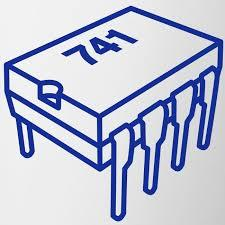# Operational Amplifier And 555 Timer A2 And A4

10 Questions | Attempts: 111
ShareSettings.

• 1.
Pick all incorrect statements. Ideal characteristics of Op-Amp are A. Open loop gain is infinite. B. Output Impedance is infinite. C. Input Impedance is Zero. D. Input Offset current is the algebraic difference between input currents.
• A.

A, B and D

• B.

B, C and D

• C.

B and C

• D.

B and D

• 2.
Find the gain of the inverting amplifier shown below. Consider R1 = 40kΩ and RF = 400kΩ Assume op-amp is ideal.
• A.

-10

• B.

10

• C.

-11

• D.

Option 4

• 3.
Open loop gain of an operational amplifier is
• A.

2*10^5

• B.

1

• C.

0

• D.

Infinite

• 4.
In the open loop configuration of OP-AMP  _____________ feedback is used
• A.

Positive

• B.

No

• C.

Negative

• D.

Series

• 5.
In given circuit Vin = 4 V, R1 = 1KΩ and R2 = 10KΩ.What is the output voltage of an amplifier
• A.

11

• B.

-44

• C.

44

• D.

-11

• 6.
For first order butterworth low pass filter, calculate cut off frequency if R=10kΩ and C = 0.001. Also calculate Avf if Rf = 100K Ω and R1 = 10kΩ.
• A.

15915 Hz,-11

• B.

15.915KHz,-11

• C.

15.915KHz,11

• D.

15.915MHz,-11

• 7.
Output produce by an op-amp is in phase when input is applied to
• A.

Inverting terminal

• B.

Non Inverting terminal

• C.

Both inverting and non-inverting terminals

• D.

Common mode

• 8.
Determine closed loop voltage gain of an non inverting amplifier if feedback resistor is double times input resistor
• A.

3

• B.

6

• C.

4

• D.

-3

• 9.
Inverting configuration adder can be used as
• A.

Summing amplifier

• B.

Averaging amplifer

• C.

Scaling amplifier

• D.

All of three

## Related Topics

×

Wait!
Here's an interesting quiz for you.